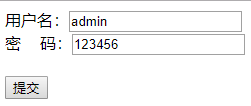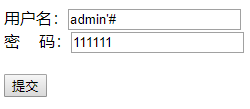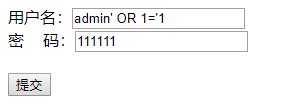# PHP SQL注入攻击与防御

PS：下章节我会就XSS/CSRF写一篇文章，还请各位关注

1.什么是SQL注入攻击？

2.PHP通用SQL注入攻击方式①首先，我们使用正确的登录用户名及密码：②我们通过SQL注入方式跳过密码验证登录：# step 1 SQL
# step 2 SQLSQL语句如下：

SELECT * FROM users WHERE username = 'admin' OR 1='1' AND password = '96e79218965eb72c92a549dd5a330112'

function addslashes_deep($value) { if (empty($value))
{
return $value; } else { return is_array($value) ? array_map('addslashes_deep', $value) : addslashes($value);
}
}

if(!get_magic_quotes_gpc())
{
$_POST = addslashes_deep($_POST);
$_GET = addslashes_deep($_GET);
}

SELECT * FROM users WHERE username = 'admin\' OR 1=\'1' AND password = '96e79218965eb72c92a549dd5a330112'

③数字型SQL注入攻击

$id =$_GET['id'];
$sql = "SELECT * FROM users WHERE id=".$id;
$res =$db->query($sql)->fetch_all(); /test.php?id=1 UNION SELECT 1,username,password,4,5,6,password,8,9,10,11 FROM users 浏览器地址栏输入如上地址，后台显示SQL如下： SELECT * FROM users WHERE id=1 UNION SELECT 1,username,password,4,5,6,password,8,9,10,11 FROM users 我们通过这里很容易利用UNION命令，查询出用户名和密码等敏感信息 当然，此类防御也相当简单，对于整数型数据，我们在获取时，需要对其进行转化如下： $id = intval($_GET['id']) 即可避免此类上述SQL注入 3.总结 通过以上两个示例，在PHP中，可以通过简单的三种方法来防御SQL注入： ①对用户的输入进行过滤处理后，在进行操作，如：addslashes()方法或者开启magic_quotes_gpc方法 ②针对获取的数值型数据，进行二次转换，如intval()，floatval() ③所有需进行数据库查询的变量都使用单引号（'）包围，如下： $sql = "SELECT * FROM users WHERE username='".$username."'"; 4.代码： 后台test.php function pretty_print($array)
{
echo '<pre>';
print_r($array); echo '</pre>'; } function addslashes_deep($value)
{
if (empty($value)) { return$value;
}
else
{
return is_array($value) ? array_map('addslashes_deep',$value) : addslashes($value); } } if(!get_magic_quotes_gpc()) {$_POST = addslashes_deep($_POST);$_GET = addslashes_deep($_GET); }$db = mysqli_connect('127.0.0.1','root','root','mysqli_test');

$username =$_POST['username'];
$password =$_POST['password'];

$sql = "SELECT *". " FROM users". " WHERE username = '".$username.
"' AND password = '".md5($password)."'";$res = $db->query($sql)->fetch_row();

if(empty($res)) { echo '登录失败，用户名或密码不正确！'; } else { echo '登录成功，欢迎您：'.$res;
}

<!DOCTYPE html>
<html>
<title>表单提交测试</title>
<meta charset="utf-8">
<body>
<form method="post" action="test.php">
<button type="submit">提交</button>
</form>
</body>
</html>

BLOG：https://www.cnblogs.com/joshua317/articles/3939718.html